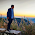## 1. What is the use of feedback amplifiers instead of conventional amplifiers?

To overcome the Drawbacks of the conventional amplifiers we used a feed back amplifiers.
Drawbacks of conventional amplifiers:
1. Gain is unstable due to the variations of transistor parameters which vary with temperature and aging of the transistor.
2. Distortion is more.
3. Effect of noise is more.
4. Desired input and output resistance cannot be achieved.

## 2. What are the Different Types of distortion present in amplifiers and explain them?

There are three types of Distortion exist in amplifiers
1. Harmonic distortion
2. Frequency distortion
3. Phase shift Distortion
Harmonic distortion: It arises due to the non linear characteristics of transistors.It mainly occurred in power amplifiers or large signal amplifiers.Due to non linear characteristics equal change in input signal do not produce proportionality equal changes in output signal due to which output signal get distorted.
Frequency distortion:Various frequencies present in the input signal gets amplified differently, if the amplifier gain is a function of frequency. This unequal amplification of various frequencies is called Frequency distortion.
Phase shift Distortion: If different frequencies present in the input signal receive unequal phase shifts then amplifier is said to have added phase shift distortion.

## 3. What are the different types of feed backs?

Feedback is nothing but it is a process of returning some part of output signal to the input of the amplifier.
There are two types of feedbacks:
1. Negative feedback
2. Positive feedback
Negative feedback: If feedback signal(returned signal) is in such a way that it reduces net input is called –ve feedback.
Positive feedback: If feedback signal is in such a way that it assists the net input  is called +ve feedback.

## 4. What is the solution to overcome the distortions in amplifiers?

By using Negative feedback

## 5. Application of Voltage Series feedback amplifiers?

source follower (which is called as the drain characteristics) and emitter follower (where the input impedance is high whereas the output impedance is low which is good for frequency response) from which the feedback gain, input resistance and output resistance is determined.

#### 1 comment :

1.Why we use +ve feedback in inverters?